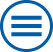## The group contributes to the following mathematical research topics of WIAS:

Analysis of Partial Differential Equations and Evolutionary Equations

Partial differential equations form an adequate and powerful instrument to provide a mathematical model for nature. At the Weierstrass Institute this research has two essential focuses: (a) Regularity for the solutions of linear elliptic equations and (b) Existence, uniqueness and regularity for evolution equations. [>> more]

Free boundary problems for partial differential equations

Free boundary problems are investigated in connection with energy technology and coating of surfaces. [>> more]

Hysteresis operators and rate-independent systems

Time-dependent processes in physics, biology, and economics often exhibit a rate-independent input-output behavior. Quite often, such processes are accompanied by the occurrence of hysteresis phenomena induced by inherent memory effects. There are two methods to describe such processes. 1. In order to describe the current state, one can add internal variables to the observable variables, and describes the evolution of internal variables. 2. For many cases one can find hysteresis operators directly describing the input-output-behavior, such that the state depends on the history of the considered process. [>> more]

Multi scale modeling and hybrid models

Because of the ongoing miniaturization, modern devices in mechanics, electronics, or optics become smaller and smaller. Their working principles depend more and more on effects on different spatial scales. The aim is to optimize the efficiency by adapting the arrangement of interfaces or periodic microstructures. The understanding of the transfer between different scales relies on mathematical methods such as homogenization, asymptotic analysis, or Gamma convergence. The generated effective models are coupled partial differential equations combining volume and interfacial effects. [>> more]

Systems of partial differential equations: modeling, numerical analysis and simulation

The mathematical description of many scientific and technological problems leads to systems of partial differential equations (PDEs). [>> more]

Variational methods

Many physical phenomena can be described by suitable functionals, whose critical points play the role of equilibrium solutions. Of particular interest are local and global minimizers: a soap bubble minimizes the surface area subject to a given volume and an elastic body minimizes the stored elastic energy subject to given boundary conditions. [>> more]